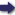(London :  Kegan Paul, Trench, Trübner & Co.,  1910.)

 Tools

## Search this bookPrev Page 116 Next``` ii6 ALBERUNTS INDIA. Rules for the compu¬ tation of the po.rvan from the Khan- dakhddyaka. Page 259. Quotation from Vara¬ hamihira's Samhitd, chap. V. 236. The computation of the pctrvan in which you happen to be is the following, according to the Khanclakhddyaka: " Write down the cthctrgctnct, as computed according to this canon, in two places. Multiply the one by 50, and divide the product by 1296, reckoning a fraction, if it is not less than one-half, as a whole. Add to the quotient 1063. Add the sum to the number written in the second place, and divide the sum by 180. The quotient, as consisting of wholes, means the number of complete parvans. Divide it by 7, and the remainder under 7 which you get means the distance of the particular parvan from the first one, i.e. from that of Brahman. However, the remainder under 180 which you get by the division is the elapsed part of the parvctn in which you are. You subtract it from 180. If the remainder is less than 15, a lunar eclipse is possible or necessary ; if the remainder is larger, it is impossible. Therefore you must always by a similar method compute that time which has elapsed before the particular parvan in which you happen to be." In another passage of the book we find the following rule: "Take the kctlpa-ctharganct, i.e. the past portion of the days of a kalpa. Subtract therefrom 96,031, and write down the remainder in two different places. Subtract from the lower number 84, and divide the sum by 561. Subtract the quotient from the upper number and divide the remainder by 173. The quotient you disregard, but the remainder you divide by 7. The quo¬ tient gives parvctns, beginning with Brcthmddi" (sic). These two methods do not agree with each other. We are under the impression that in the second pas¬ sage something has either fallen out or been changed by the copyists. What Varahamihira says of the astrological portents of the parvans does not well suit his deep learning. He says: "If in a certain parvan there is no eclipse, but there is one in the other cycle, there are no rains, ```Prev Page 116 Next